# Transition words in reading and writing

##By Snap Language

Transition words help writers connect ideas between sentences and paragraphs. When you read, transitions help you understand not only the ideas themselves but also the relationship between them.# Antonyms and Synonyms

##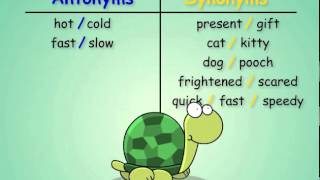By Vocabulary SpellingCity

Word relationships can be tricky, but understanding them is key to really understanding language. VocabularySpellingCity.com can help! This video lesson explains a little bit about Antonyms and Synonyms.Learn more at http://www.spellingcity.com/synonyms-and-antonyms.htmlSpellingCity has both a free and premium service, the free service on VocabularySpellingCity allows teachers and parents to register and save lists. The premium version of VocabularySpellingCity gives access to the vocabulary and writing materials, the best spelling games, and give students their own login.# Area of an equilateral triangle | Perimeter, area, and volume | Geometry | Khan Academy

##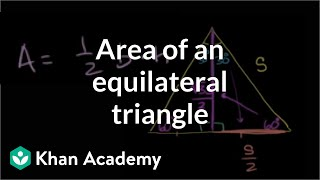By Khan Academy

Finding the formula for the area of an equilateral triangle with side s. All Khan Academy content is available for free at www.khanacademy.org# The converse of the Pythagorean theorem and special triangles

##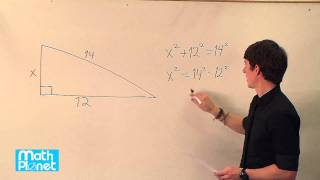By MathPlanetVideos

Find the value of x in the right triangle.# Pythagorean theorem 3 | Right triangles and trigonometry | Geometry | Khan Academy

##By Khan Academy

This three-minute video is from Sal Khan about using the Pythagorean Theorem to solve a word problem: A carpet measures 7 feet long and has a diagonal measurement of square root of 74 feet. Find the width of the carpet Mr. Khan uses computer software for demonstration. The screen is a little 'busy' so the viewer may want to open the screen to 'full screen' to see all more clearly.# Word Problem Involving the Perimeter of a Rectangle – Ex 1

##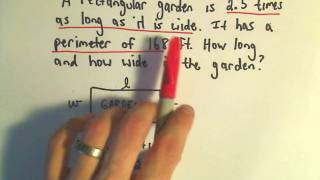By PatrickJMT

YouTube presents Word Problem Involving the Perimeter of a Rectangle, an educational video resource on math.# Proof of Pythagorean Theorem using similar triangles

##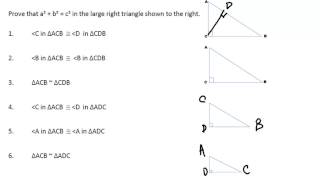By Matt Stryker

Proof of Pythagorean Theorem using similar triangles# Word Problem Involving Perimeter of a Triangle - ex 2

##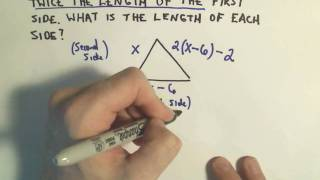By PatrickJMT

YouTube presents Word Problem Involving Perimeter of a Triangle - ex 2, an educational video resource on math.# Triangle inequality theorem | Perimeter, area, and volume | Geometry | Khan Academy

##By Khan Academy

Salman Khan offers his intuition behind the triangle inequality theorem during this six-minute video lesson.# Another Pythagorean theorem proof | Right triangles and trigonometry | Geometry | Khan Academy

##By Khan Academy

Khan Academy presents Another Pythagorean Theorem Proof, an educational video resource on math.# Pythagorean theorem 1 | Right triangles and trigonometry | Geometry | Khan Academy

##By Khan Academy

This is a Khan Academy video about solving a word problem involving the need to find the length of a rope that attaches a mast of a boat with the deck of the boat.# Pythagorean theorem 2 | Right triangles and trigonometry | Geometry | Khan Academy

##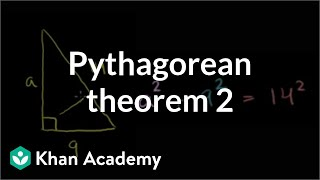By Khan Academy

In this video, Sal Khan continues discussing the Pythagorean Theorem to solve for the third side of the triangle Mr. Khan uses computer software for demonstration. The screen is a little 'busy' so the viewer may want to open the screen to 'full screen' to see all more clearly.# More ways to think about multiplying | Multiplication and division | 4th grade | Khan Academy

##By Khan Academy

When multiplying, it can be helpful to break numbers into parts.# Inverse Functions | MathHelp.com

##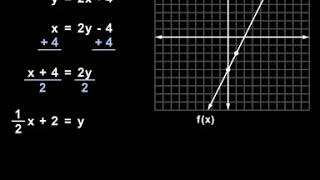By MathHelp.com

In this example, we’re given a relation in the form of a chart, and we’re asked to find the inverse of the relation, then graph the relation and its inverse. To find the inverse of a relation, we simply switch the x and y values in each point. In other words, the point (1, -4) becomes (-4, 1), the point (2, 0) becomes (0, 2), the point (3, 1) becomes (1, 3), and the point (6, -1) becomes (-1, 6). Next, we’re asked to graph the relation and its inverse, so let’s first graph the relation. Notice that the relation contains the points (1, -4,), (2, 0), (3, 1), and (6, -1). And the inverse of the relation contains the points (-4, 1), (0, 2), (1, 3), and (-1, 6). Finally, it’s important to understand the following relationship between the graph of a relation and its inverse. If we draw a diagonal line through the coordinate system, which is the line that has the equation y = x, notice that the relation and its inverse are mirror images of each other in this line. In other words, the inverse of a relation is the reflection of the original relation in the line y = x.# The Pythagorean theorem intro | Right triangles and trigonometry | Geometry | Khan Academy

##By Khan Academy

Watch this video to review right triangles and the Pythagorean Theorem view an example of using the Pythagorean Theorem to find the length of a hypotenuse (the longest side of a right triangle) view an example of using the Pythagorean Theorem to find the length of a leg of a right triangle# Inverse Relations | MathHelp.com

##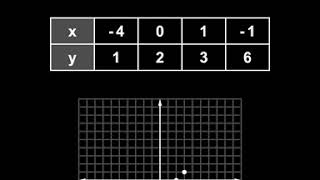By MathHelp.com

Here we’re asked to solve for x in the equation: log base x of 144 = 2. Notice that we have a logarithmic equation, so let’s first convert the equation to exponential form. Remember that the base of the log represents the base of the power, the right side of the equation represents the exponent, and the number inside the log represents the result, so we have x…squared…= 144. Now, to solve for x, since x is squared, we simply take the square root of both sides of the equation to get x = plus or minus 12. Remember to always use plus or minus when taking the square root of both sides of an equation. However, notice that x represents the base of the logarithm in the original problem, and the base of a logarithm cannot be negative. Therefore, x cannot be equal to negative 12. So our final answer is x = 12.# Lesson 9 - Synonyms for Love - English Vocabulary

##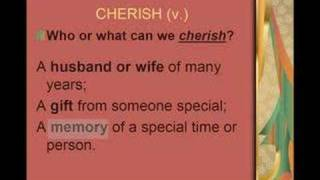By JenniferESL

Another lesson in honor of Valentine's Day. This lesson demonstrates how knowing synonyms for common words can enrich your vocabulary, giving you greater accuracy and variety. The speaker uses sentences with love in them and shows how to find a different word-synonym for love examples-admire, cherish, and adore.This video does a great job in defining synonyms for love, using the new words in sentences, and providing practice exercises# Math Antics - What Percent Is It?

##By mathantics# Math Antics - The Pythagorean Theorem

##By mathantics# Math Antics - Circles, What Is PI?

##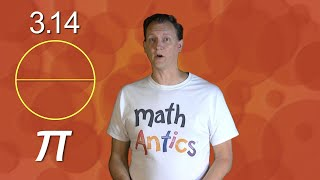By mathantics